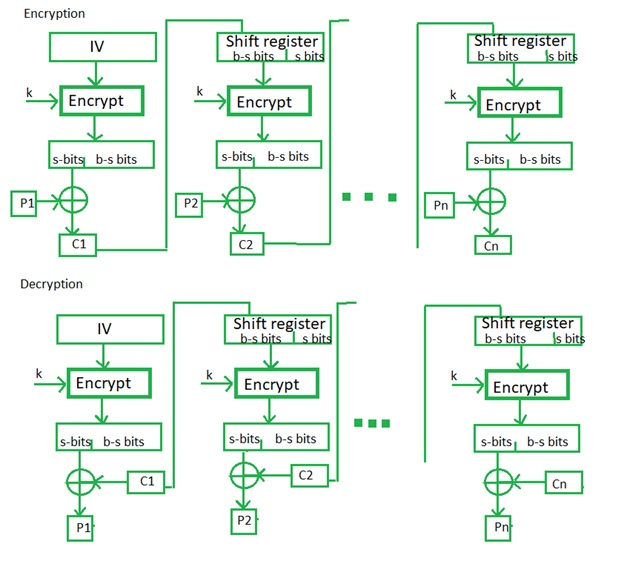# Ciphertext Feedback (CFB) in Cryptography

Cryptography | Ciphertext Feedback (CFB): In this tutorial, we will learn about ciphertext feedback, its operation, and its advantages. By Monika Sharma Last updated : May 25, 2023

## Ciphertext Feedback (CFB)

This is Ciphertext feedback (CFB) which is also a mode of operation for a block cipher. In contrast to the cipher block chaining(CBC) mode, which encrypts a set number of bits of plaintext or original text at a time, it is at times desirable or sensible to encrypt and transfer or exchange some plaintext or original text values instantly one at a time, for which ciphertext feedback is a method in cryptography. Like cipher block chaining(cbc), ciphertext feedback(cfb) also makes use of an initialization vector (IV) in the blocks. CFB uses a block cipher as a component of a different or random number generator in this. CFB mode, the previous ciphertext block is encrypted and the output is XORed (see XOR) with the current plaintext or original text block to create the current ciphertext block from this. The XOR operation conceals plaintext or original text patterns. Original text or plaintext cannot be directly worked on unless there is the retrieval of blocks from either the beginning or end of the ciphertext in the cryptography.

This is entropy that results can be implemented or work as a stream cipher. CFB is primarily a mode to derive some characteristics of a stream cipher from a block cipher in the cryptography in cryptoanalysis. In common words with CBC mode, changing the IV to the same plaintext or original text block results in different outputs. Though the IV need not be secret, some applications would see this desirable or wise into this. This is Chaining dependencies are similar to CBC in the cryptography, in that reordering ciphertext block sequences alters decryption output, as decryption of one block depends on the decryption of the preceding blocks in the cryptography.

In this mode the cipher is given as feedback from the block to the next block of encryption with some new specifications of this feedback: first, an initial vector as an IV is used for first encryption and output bits from character are divided as set of s and b-s bits the left-hand side s bits are selected and are applied an XOR operation with plaintext or original text bits. The result is given as input to a shift register in the cryptography and the process continues. The encryption and decryption process for the same is shown below, both of them use encryption algorithm in this.

## Ciphertext Feedback (CFB) Operation

The operation of CFB mode is depicted in the following illustration or steps. For example, like, in the present system, a message block has a size ‘s’ bits of character where (1 < s < n). The CFB mode requires an initialization vector (IV) as the initial random n-bit input block of the character. The IV need not be secret in this.

Steps of operation are,

• Firstly, Load the IV in the top register.
• Then, Encrypt the data value in the top register with the underlying block cipher with key K to the block.
• Then, take only 's' number of most significant bits as left bits of the output of the encryption process and XOR them with 's' bit plaintext or original text message block to generate ciphertext block in cryptography.
• This, Feed ciphertext block into the top register by shifting already present data to the left and continue the operation till all plaintext or original text blocks are processed in this mode.
• Essentially, the previous ciphertext block is encrypted with the key, and then the result is XORed to the current plaintext block or original text.
• Similar steps are followed for decryption cryptography. Pre-decided IV is initially loaded at the start of decryption in the cryptography.Image source: https://www.geeksforgeeks.org/block-cipher-modes-of-operation/

Since, in this, there is some data loss due to the use of shift register of the block, thus it is difficult for applying cryptanalysis in the cryptography.